# The loss of speed in the series

## The loss of speed in the series and its relationship with ammonia and lactate

At this point, the article analyzes the effect of losing speed in the series of repetitions within strength training.

In this series of articles we deal with some of the most important concepts of strength training, collecting notes from the recently published book Strength, Speed ​​and Physical and Sports Performance written by renowned researchers Juan José González Badillo and Juan Ribas Serna.

### SUMMARY

• The loss of speed in the series can serve as a predictor of the degree of metabolic stress caused by training, and therefore it is a good indicator to estimate fatigue.
• Doing half or less of the repetitions achievable in the series produces notable improvements in muscular strength and sports performance.
• People who train for improved health should not do even half of the possible repetitions in the series.
• Most experienced athletes with medium-high strength needs will probably be able to perform at most half or 1-2 repetitions more than half of the possible ones.
• A subject should not lose more than 20-35% (depending on each exercise) of the speed of the first repetition in the series.

This is the second question related to the definition of the stress character (CE) as a solution to the problems raised by RM and XRM. Speed ​​control not only makes it possible to know very precisely the true effort that a given load (mass) represents when doing the first repetition of a series, but also allows complete knowledge of the degree of effort made by knowing in what proportion or percentage speed is lost as repetitions are made within the series.

And this is important because the loss of speed is a highly valid indicator to estimate fatigue (Edman, 1992; Allen, Lamb, 8 Westerblad, 2008). This validity is based on the high relationship that exists between the loss of speed in the series and the loss of speed at a certain absolute load measured before and immediately after making the effort.

#### speed loss is a highly valid indicator to estimate fatigue

In turn, the loss of speed in the series can serve as a predictor of the degree of metabolic stress caused by training. Indeed, Sánchez-Medina and Gonzalez-Badillo (2011) carried out a study with 15 types of effort in the bench press and squat, with loads that could be done between 12 and 4 repetitions per series. These intensities correspond to mean relative intensities between 70 and 90% of the RM, although each subject did not really make the effort exactly with said intensities, but with the absolute loads with which they could do the marked repetitions.

The absolute loads used were those with which 12, 10, 8, 6 and 4 maximum repetitions could be done, which, on average, corresponds to relative intensities of 70, 75, 80, 85 and 90% of the RM. , respectively. The greatest effort with each load consisted of doing three series with the maximum number of repetitions possible (or one less than possible in the first series) and the least effort in doing three series with half the possible repetitions.

In addition, one or two more efforts were made with an intermediate number of repetitions. For example, with the load that 12 repetitions could be done, four efforts were made, performing three series of 12, 10, 8 and 6 repetitions in the series, which were represented as follows: 3×12(12), 3×10(12) , 3×8(12) and 3×6(12).

In total, 15 efforts were made with each of the exercises: bench press and squat. The valuation of The degree of fatigue generated with each effort was determined through the loss of speed with the load that could be moved at 1 m*s-1 before making the effort., as well as the loss of jump (really loss of execution speed) pre-post effort when the squat exercise was performed.

Before starting the squat training, the vertical jump test (CMJ) was performed after a specific warm-up. In both exercises, the warm-up began with progressive loads and when passing through the load that could be moved at approximately 1m*s-1, three repetitions were performed with it and the value of the load and the concrete average speed of the three repetitions were noted immediately after making the effort, the measurement was made again. jump (after the squat) and the load of 1 m*s-1 in both exercises.

Since the minimum load with which the efforts were made was approximately 70% of the RM, an approximate load of 60% was always used in the squat during the warm-up (load that moves approximately 1 m*s-1). and 45% in bench press (load that moves approximately 1 m*s-1).

In addition, after each effort, lactate and ammonium levels were measured. In figure 1 you can see the scheme of the execution of the efforts and the initial and final tests, in this case in the bench press exercise and with the load that could be done 12 repetitions: 3 series of 12 repetitions being able to make 12: 3×12(12).

In this case, the average speed before the effort with the load of 1 m*s-1 was 1.03 m*s-1. The subject continues his warm-up until he reaches the load with which he has to carry out the effort of the day: 3×12(12) and performs the 3 series at the maximum possible speed, with 5 minutes of recovery between series.

The speed with each repetition in the three series is represented by the three groups of central bars with a tendency to decrease. Immediately after (10-15 s) the last repetition of the last series, the load with which the speed of 1 m*s-1 was initially reached was measured again. In this case, the final average speed of the three repetitions was 0.71 m*s-1. The loss of speed, in this case 31.1%, reflects the quantification of fatigue.

#### The loss of speed, in this case 31.1%, reflects the quantification of fatigue.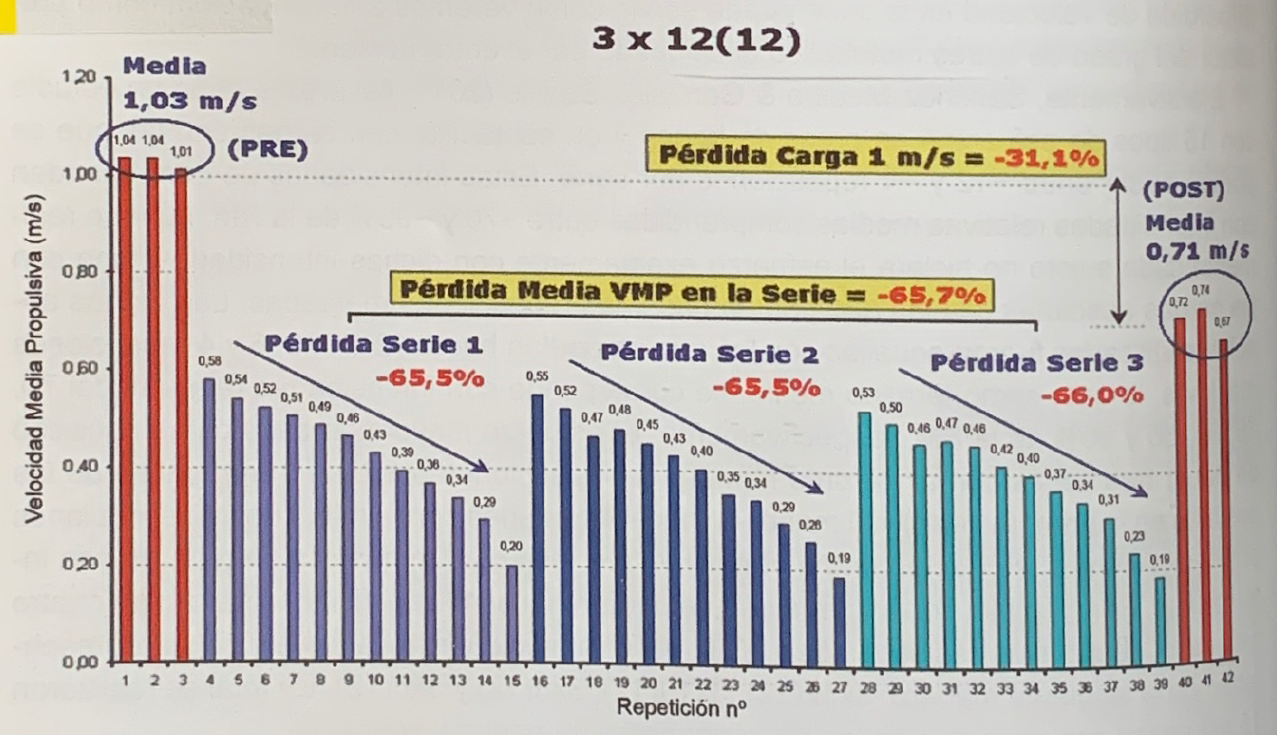Figure 1. Outline of the protocol followed in an effort of 12 repetitions, being able to do 12: 3×12(12), in the bench press exercise. Red bars, speed with the load of 1 m*s-1 before and after making the effort. Rest of the bars: speed with each repetition in the three series performed with the expected load (Sánchez-Medina and González-Badillo. Med. Sci. Sports 2011)

As a result of this study, high relationships were found between the loss of speed in the series and the loss of speed with the load that moved 1 m*s-1 before the effort, both in the bench press (r = 0, 97) as in the squat (r = 0.91), and with the loss of height (loss of speed) in the jump after the squat (r = 0.92). These results confirm that the greater the speed loss in the series, the greater the fatigue.

Later on, it will be analyzed more precisely how the degree of fatigue (loss of speed with the load of 1 m*s-1 and loss of jump) is dependent on the speed of the first repetition (real percentage of the RM) and of the series loss. Likewise, high curvilinear relationships were found between the speed loss in the series, the jump loss and ammonium [R2 = 0.89 in the bench press; R2 = 0.85 in the squat and R2 = 0.86 in the CMJ (figure 2).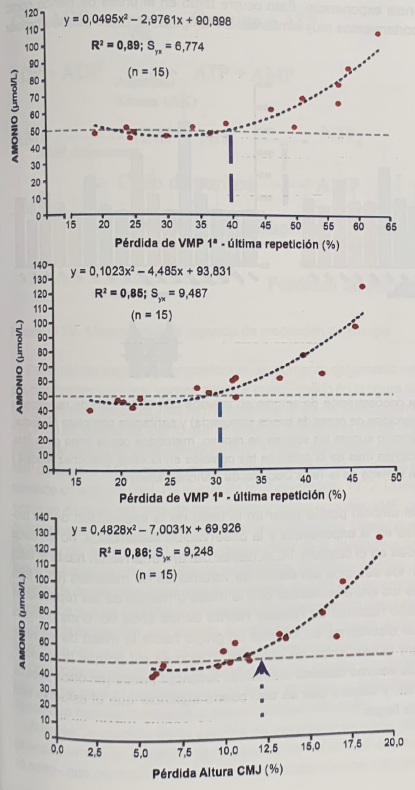Figure 2. Relationship between velocity loss and ammonium concentration with bench press (top figure) and squat (middle figure) exercises, and relationship between vertical jump losses after squat exercise and ammonium concentration (bottom figure). ). Note that from an approximate loss of speed in the series of 40% in the bench press, 30% in the squat, and 12% in the vertical jump, the ammonium concentration shoots up. (Sánchez-Media y González-Badillo, 2011)

An important and unique observation to date is that for an increase in ammonia to occur it was necessary to perform 1-2 repetitions more than half the number possible at any load and in both exercises. This can be seen in figure 3. The horizontal dotted line represents the baseline ammonium value. Only when more than half of the possible repetitions in the set are performed does the ammonium spike with an exponential trend.

#### For an increase in ammonium to occur, it was necessary to perform 1-2 repetitions, more than half of those possible, at any load.

This occurs in both the bench press and the squat, with very similar behaviors. Figure 2 also shows this trend.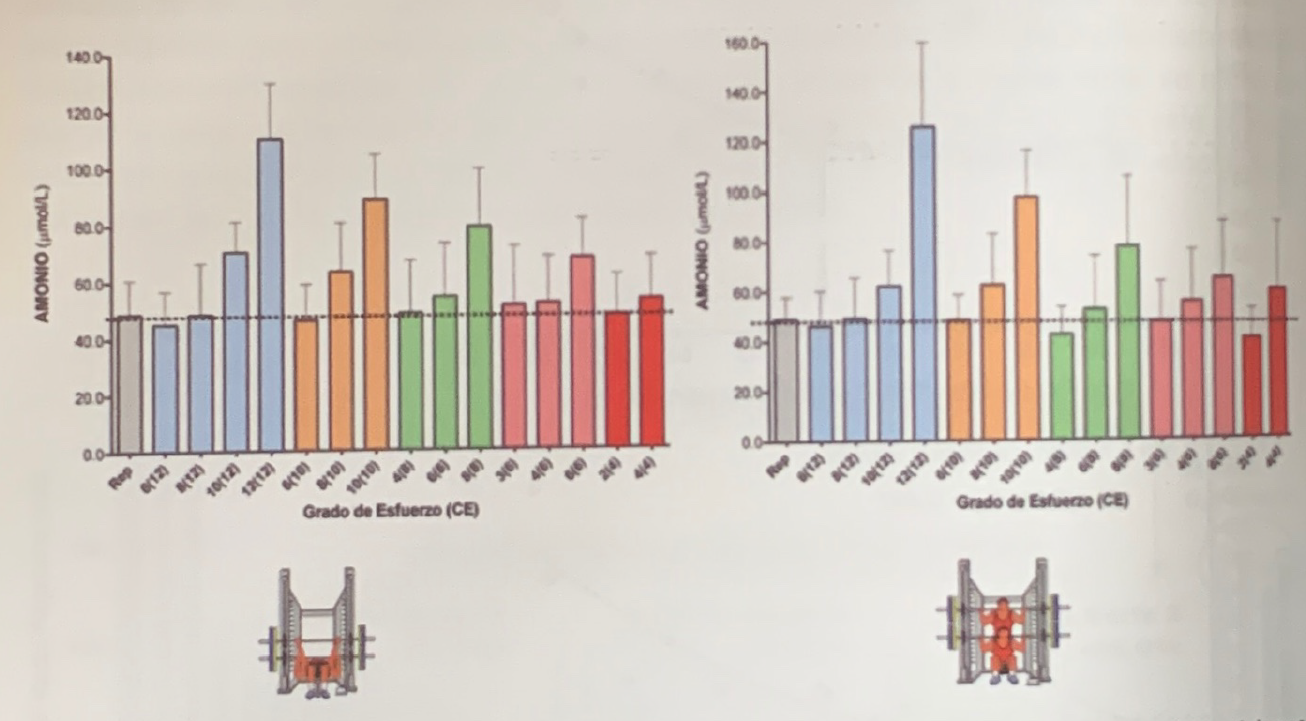Figure 3. Evolution of ammonium concentration in relation to the number of repetitions performed in the series with the bench press (left) and right full squat exercises. It is observed that for the ammonium to exceed the resting values, marked by the dotted line, it is necessary to do 1-2 repetitions, more than half of those possible in the series (Sánchez Medina and González Badillo, 2011). Figure taken from Sánchez-Medina’s Doctoral Thesis.

This behavior of ammonium could be at the base of the explanation of the proposals or hypotheses (based on experience and systematic observation, not on experimental data, when speaking of training to failure).) made in the 80s, with studies of the effect of non-maximal volumes (65 and 85% of the maximum achievable), or of training with half or less of the possible repetitions in the series of the National Field Hockey team of the 90s, or the first experimental studies that were designed in which one group did half the repetitions possible in the series and the other all the possible ones.

#### the appearance of ammonium above the basal values when lifting weights may mean that the effort is at the limit that should be reached.

And this is so because the appearance of ammonium above the basal values when lifting weights (in other types of exercises it may be different, and it certainly is) may mean that the effort is at the limit that should be reached.

The measurement of the loss of speed in each repetition and the degree of fatigue generated -measured through the loss of speed with the load of 1 m*s-1 and loss of jump- allow us to add much more precise information about this behavior. of ammonium than the simple count of the repetitions performed.

These speed losses in the series from which the ammonium is fired correspond to certain speed losses with the 1 m*s-1 load and height loss in the jump. The data with the following:

• With a loss of 40% speed in the bench press, ammonium is triggered (figure 2) and would correspond to a loss of speed of 17% with a load of 1 m*s-1.
• With a 30% loss of speed in the squat, ammonium is triggered and would correspond to a loss of speed of 12.5% with the load of 1 m*s-1.

With a 12% loss in jump height, ammonium shoots up and would correspond to a loss of speed in the series of 32% (Sánchez-Medina and González-Badillo, 2011).

It can be observed how the same fatigue generated when performing the squat, with 30 and 32% loss of speed in the series, is estimated in an equivalent way by the loss of speed with the load of 1 m*s-1 (12.5 %) and height in the jump (12%), respectively. This indicates that the loss of speed is an accurate indicator of fatigue, since its quantification before the same effort (loss of 30-32% of speed in the squat) can be done at different speeds, giving practically identical results.

In this case, the initial speed of 1 m*s-1 in the squat and the speed of the vertical saint have been used, which, on average, is performed at a clearly higher average speed, which could be approximately more than 1.5 m-s. * on average, which would be equivalent to a little more than 45 cm of initial jump.

If now the two variables used are analyzed: the loss of speed in the series and the number of repetitions performed, it can be confirmed that in the bench press exercise the loss of speed when half of the possible repetitions has been done is between 25 and 30% (González-Badillo et al., 2017) of the speed of the first repetition, that is, slightly below the loss caused by the ammonium shot, and that in the complete squat the loss of speed when doing half of the possible repetitions it would be approximately 15-20% (Rodríguez-Rosell et al., 2019), that is, also below the loss of speed caused by the increase in ammonia.

Therefore, if it is known what degree of effort (degree of fatigue) means each percentage of speed loss in the series, the application of speed as a training control method is very useful, it is probably the best procedure to estimate with high precision and immediately the training load.

#### ammonium concentration above quiescent values can be controlled by the loss of speed in the series

This load would be determined by the degree of fatigue caused by the joint effect of the volume and intensity used in training. Therefore, the ammonium concentration above the resting values can be controlled by the loss of speed in the series, since there is a close relationship between the loss of speed in the series and the percentage of repetitions performed (González-Badilo et al, 2017; Rodriguez-Rosell et al., 2019).

If, furthermore, it is known, through extensive practical experience, that doing half or less of the repetitions achievable in the series produces notable improvements in muscular strength and sports performance, it would not be very advisable to frequently exceed (in some cases it would never be necessary) half of the repetitions that can be done in a series. This practical experience has been reinforced by experimental studies in which it has been proven that losing 10-20% of the speed in the series, which is equivalent to performing half or less of the possible repetitions in the series, in the exercise of squat offers better results than losing 30-40%, which leads to a situation at the limit of ammonium increase (30% loss) or very close to muscle failure (40%) (Pareja-Blanco et al., 2017 ; Rodríguez-Rosell, Doctoral Thesis).

In addition to the relationship with ammonium, velocity loss also showed high positive linear correlations with lactate concentration: [r = 0.95 in the bench press, r = 0.97 in the squat, and r = 0.97 in the the jump (figure 4)].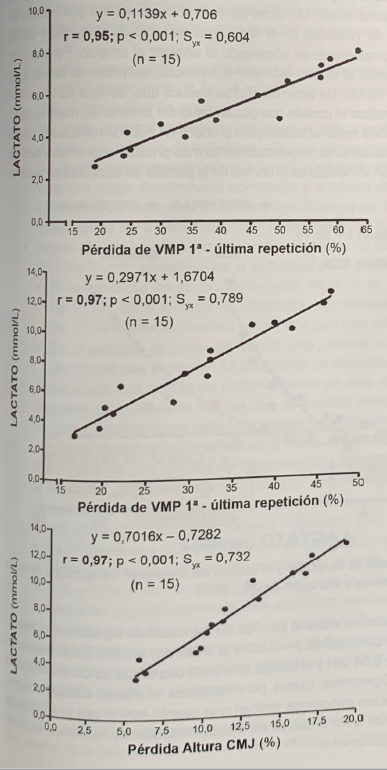Figure 4. Relationship between velocity loss and lactate concentration with bench press (upper figure) and squat (middle figure) exercises, and relationship between vertical jump losses after squat exercise and lactate concentration (lower figure). ). (Sánchez-Medina and González-Badillo, 2011).

#### Doing half or less of the repetitions achievable in the series produces notable improvements in muscular strength and sports performance.

If the regression equations corresponding to each of the relationships of the three exercises with lactate are applied, it is verified that before a 40% loss of speed in the bench press series, which is when ammonium is triggered, the lactate would be 5.3 mmol/L, in the squat, when ammonium increases, when losing 30% of the speed, lactate would be 7.2 mmol/L, and in the jump, when 12% is lost jump and the ammonium increase begins, the lactate would be 7.7 mmol/L.

As can be seen, the height loss in the vertical jump (12% jump loss and 32% speed loss in the squat) when ammonium is fired it corresponds to practically the same concentration of lactate than when ammonium is triggered by the loss of speed in the series in the squat exercise (30%).

Which corresponds to the behavior observed when analyzing ammonium. Therefore, ammonium begins to rise when lactate is 5.3 mmol/L in the bench press and 7.2 mmol/L in the squat (figure 15.14). From this relationship it can be deduced that, although it is not the most practical and viable option, if one wanted to analyze the possible behavior of ammonium without measuring it, given its greater difficulty and price, one could measure lactate to predict at what moment the lactate begins to be triggered. ammonium.

Although, naturally, the most precise, economical, easy-to-perform procedure and with immediate information is the control of speed loss in the series.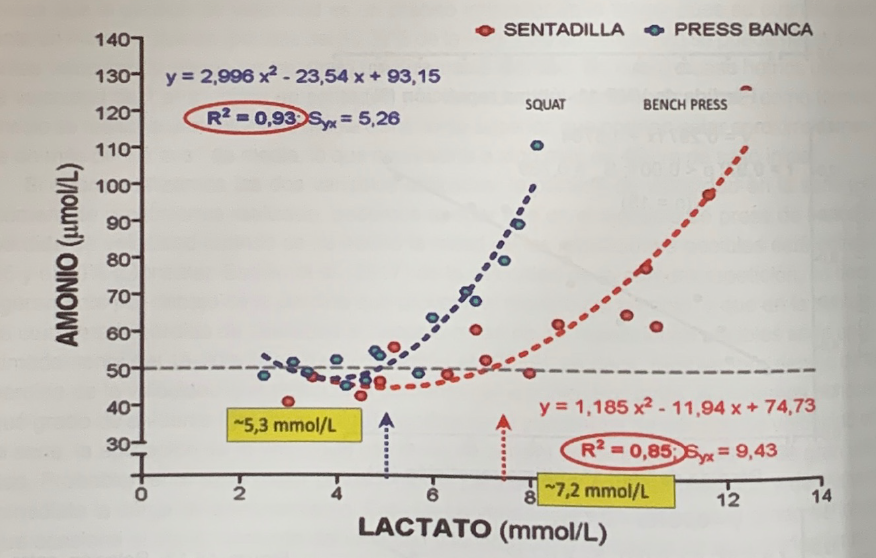Figure 5. Relationship between lactate values and ammonium firing in bench press and squat exercises (Sánchez-Medina and González-Badillo, 2011)

There were also high relationships between stalling and testosterone (r=0.83), growth hormone (r= 0.82), and insulin (r= 0.88). These relationships increased for ammonium (p = 0.94-96) and lactate (p = 0.98) when Spearman’s rank correlation coefficient was used (data from the same unpublished study). All these relationships indicate that the higher the speed in the series, the greater the mechanical, metabolic and hormonal stress tends to be, the greater the degree of effort generated.

#### the greater the speed loss in the series, the greater the mechanical, metabolic and hormonal stress tends to be, the greater the degree of effort generated.

The question that should be asked as a result of this knowledge is what should be the optimal loss of speed in each case. This question, of course, does not have an easy answer, but being able to ask it, and having the appropriate mechanical and physiological data available to try to find an answer, is already a great advance.

In the next articles, useful studies for the practice of training and that provide answers to many of these questions will be reviewed.

### Conclusions

From the above it can be deduced that the knowledge of the relationship between the loss of velocity in the serle and the loss of velocity with the load of 1 m*s-1 and the height of the CMJ, as well as the metabolic stress allows us to conclude the following:

• The fatigue caused by a training session of three sets with loads that allow you to do between 12 and 4 repetitions per set depends on the percentage of speed lost in the set.
• The training load can be quantified by the loss of jumping capacity and the loss of speed before a determined load (mass) in each session.
• The relationship between the loss of jump and the loss of speed could be verified before a determined load per session and the effect of the training.
• The loss of velocity in the series with the load of 1 m*s-1 and in the CMJ are accurate estimators of the metabolic stress caused by the training session.
• Depending on the metabolic stress generated, a subject should not lose more than 20-35% (depending on exercises) of the speed of the first repetition in the series:
• Performance is probably not better if you lose a higher percentage of speed. In the squat exercise, an average loss of speed in the set of 10-20% offered better results than a loss of 30-40%.
• In the bench press exercise, a mean loss of 27.7% outperformed losing 53.3% (unpublished laboratory data).

#### a subject should not lose more than 20-35% (depending on exercises) of the speed of the first repetition in the series

• If you do a training session of three sets with any load between those with which you can perform between 12 and 4 repetitions per set, performing a range of repetitions between half and the maximum of the possible repetitions in the series, ammonium increases exponentially from a loss of speed of 40% in the bench press and 30% in the squat. In the case of the vertical jump, the increase in ammonia occurs when a pre-post effort jump loss of 12% is reached.
• As a practical application of synthesis, it is suggested:
• People who train for improved health should not do even half of the possible repetitions in the series.
• Most experienced athletes with medium-high strength needs will probably be able to perform at most half or 1-2 repetitions more than half of the possible ones. Although we also estimate that athletes with lower strength needs probably, even if they are very experienced, do not need to perform even half of the possible repetitions in the series at any time.